Next: Quadratic Stark Effect Up: Time-Independent Perturbation Theory Previous: Two-State System

# Non-Degenerate Perturbation Theory

Let us now generalize our perturbation analysis to deal with systems possessing more than two energy eigenstates. The energy eigenstates of the unperturbed Hamiltonian,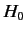, are denoted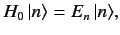(601)

where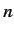runs from 1 to. The eigenkets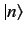are orthogonal, form a complete set, and have their lengths normalized to unity. Let us now try to solve the energy eigenvalue problem for the perturbed Hamiltonian: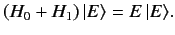(602)

We can express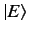as a linear superposition of the unperturbed energy eigenkets,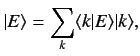(603)

where the summation is from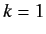to. Substituting the above equation into Equation (602), and right-multiplying by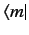, we obtain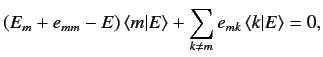(604)

where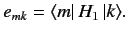(605)

Let us now develop our perturbation expansion. We assume that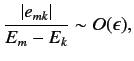(606)

for all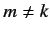, where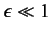is our expansion parameter. We also assume that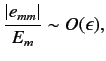(607)

for all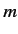. Let us search for a modified version of theth unperturbed energy eigenstate, for which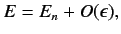(608)

and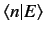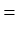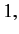(609)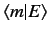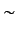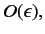(610)

for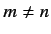. Suppose that we write out Equation (604) for, neglecting terms that are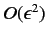according to our expansion scheme. We find that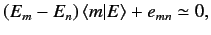(611)

giving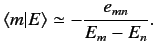(612)

Substituting the above expression into Equation (604), evaluated for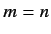, and neglecting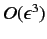terms, we obtain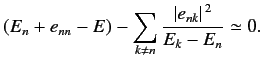(613)

Thus, the modifiedth energy eigenstate possesses an eigenvalue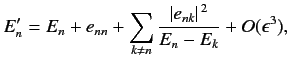(614)

and a eigenket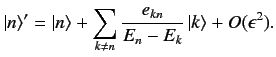(615)

Note that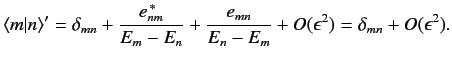(616)

Thus, the modified eigenkets remain orthogonal and properly normalized to.Next: Quadratic Stark Effect Up: Time-Independent Perturbation Theory Previous: Two-State System
Richard Fitzpatrick 2013-04-08